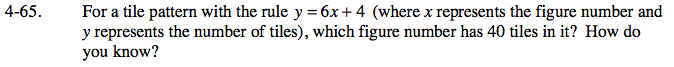### Home > CAAC > Chapter 4 > Lesson 4.1.7 > Problem4-65

4-65.Given information:
y = number of tiles
x = figure number
40 tiles

Substitute in the given information.

number of tiles = 6( figure number ) + 4

40 = 6x + 4

x = Figure 6